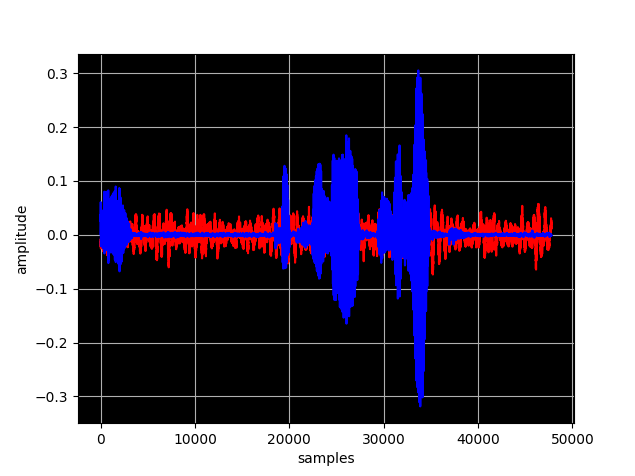# Scikits

•## Manipulating audio files using Python and Linux

Tag: , , , , , , , |

In this post we are going to manipulate some wav files using Python. We would like to add some noise or sound to a recorded wav file. Record your voice Let's record a sound sample of your voice using the rec command userk@dopamine:~$mkdir temp && cd temp userk@dopamine:~/temp$ rec voice.wav Input File :

•## Python – Scikits Two Point Boundary Value Problem

|

An application of Calculus of Variation is the determination of control action $u(t)$ such that a system described by a differential equation system $$\dot{x}(t) = {a(x(t),u(t),t)}.$$ follows a trajectory $x(t)$ for all time instants that minimizes a performance measure $J(u)$ defined by $$J(u) = \int_{t0}^{tf} {g(x(t),u(t),t)} dt$$ A wonderful application could be a tridimentional trajectory